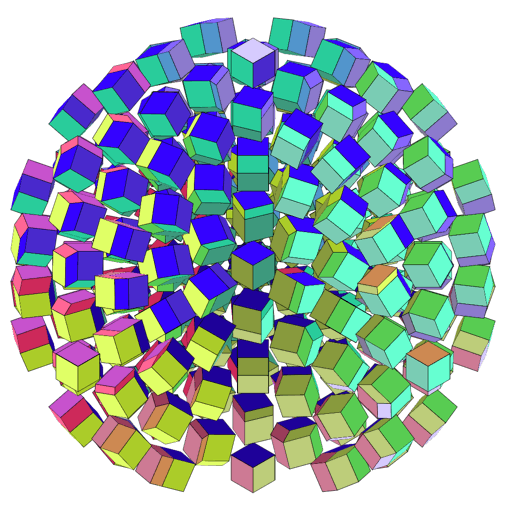SO(4) portrays the group of rotations in four dimensions, by showing the effect of various rotations on a hypercube. A hypercube generally projects down to three dimensions as an irregular rhombic dodecahedron, with half the hypercube’s 24 faces buried in the interior of the dodecahedron.

Four-dimensional Euclidean space can be equipped with a rule for multiplying vectors, forming an algebraic structure known as the quaternions. The three-dimensional surface of the four-dimensional unit sphere in the quaternions is closed under multiplication, and comprises a group known as SU(2). Left or right multiplication by a fixed element of SU(2) rotates the whole 3-sphere, and any rotation can be produced by a combination of left and right multiplication.

Clicking on the applet alternates between two different views of SO(4). In the first view, rotations are produced by right-multiplication with elements taken from one half of SU(2) — drawn here as a 3-dimensional ball, with a wedge removed to reveal the interior — and left-multiplication with elements taken from a path that wraps around another copy of the group (not shown). Traversing this path reveals a 4-dimensional swath of the whole 6-dimensional manifold. The net rotation is given by the function T(v)=LvR, and since (–L)v(–R)=LvR, using both halves of both copies of SU(2) would be redundant.

In the second view, the half-copy of SU(2) is used to produce rotations via RvR–1. These are effectively three-dimensional rotations, since they leave the identity vector unchanged. Left-multiplication with elements from a path around the other, full copy of SU(2) gives a net rotation of T(v)=LRvR–1. For each choice of L, antipodal points R and –R will have the same effect, so as the path is traversed, every slice of SO(4) shown in this view has the same topology as SO(3), the group of rotations in three dimensions.Applets Gallery / SO(4) / created Sunday, 24 September 2000